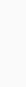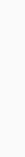Class: Pre-Nursery

Term: First, Week: 4-6

Subject: Number work

Topic: Oral counting of numbers 1-20

Writing of numbers 1-5

Recognition of numbers 1-10

## OBJECTIVES

At the end of the lesson, the pupils should be able to:

• Count numbers 1-20
• Write numbers 1-5
• Identify numbers 1-10
• Demonstrate/internalize the concept of numerical values of numbers 1-5

## PRESENTATION

The teacher delivers the lesson as in the following steps:

### Step 1: Introduction

To introduce the lesson, the writes the topic on the board and revises the previous lesson:

• Reminds the pupils the meaning of numbers: a number is what tells us how many of a thing we have.
• S/he tells them that there are many numbers because we can have many things.
• Each of the many numbers has its special name and way it is written.
• For example, they learned ten numbers in the last lessons.

After the last exposition, the teacher asks the pupils if they remember the ten numbers – they count together. Afterwards, teacher writes any number(s) between 0-5 and asks the pupils to name. Finally, the teacher asks which is greater between some given numbers.

These been done as stated, the teacher tells the pupils that they shall in the week’s lesson, learn more numbers.

### Writing Numbers 1-5

Succeeding the introduction, the teacher tells the pupils that before they learn more numbers, they have to learn how to write some of the numbers they learned in the previous lessons. Hence, picking each one at a time, the teacher analyses, demonstrates and guides the pupils to form the numbers 1-5.

#### How to Write Number One (1)

This is a single vertical stroke (refer to pre-writing pattern). To form it, the teacher makes two dots such that one is directly above the other:Then both dots are joined with a straight line:#### How to Write Number Two (2)

This number has a curve, a slant line and a horizontal line. To form number 2, start with the curve:Join the slant line:And finally the horizontal line:#### How to Write Number Three (3)

Number three is two curves joined. To form number 3, mark off three dots such that the dots are directly below the top – the middle being at the centre:Then draw a curve from the top dot to the middle:And from the middle to the bottom:#### How to Write Number Four (4)

Number four has three lines – two verticals and a horizontal. To form number 4, draw the first vertical stroke:Then from the bottom end of the vertical line, draw a horizontal:And finally draw another vertical across the horizontal:#### How to Write Number Five (5)

Number five has three strokes – a horizontal, a vertical and a curve. To form it, draw the horizontal:Then the verticalAnd finally the curve:For each number, the teacher analyses or describes the formation as given; demonstrates how to form it while the pupils do the same.

Please note that you may need to practice some few times before the pupils perfect the notation – be patient.

### Counting numbers One (1) – Twenty (20)

After assessing the pupils’ performance in writing number 1-5, the teacher applauds them and gives them feedback – This is basically telling them specifically, what they need to improve upon. Afterwards, the teacher tells them that they are now ready and going to learn more numbers – she may then first of all lead them to count 1-10. Thereafter, the teacher picks each of numbers 11 through 20, explains its value and teaches the pupils the correct pronunciation as described below:

• Tells the pupils that the next number after ten is eleven and is written as 11.
• Tells them that eleven is greater than 10 and all the numbers below 10.
• If necessary, reiterates in local dialect.
• Pronounce the number distinctively and let the pupils repeat after him/her several times.
• The teacher then gives the pupils or directs them to pick eleven counters
• Finally, the pupils count the counters all together.
• Move to next number 12, 13, 14, 15 16, 17, 18, 19, 20.

#### General counting with stand counters

After the explanation, the leads the pupils into general counting:

• S/he puts up the stand counter of 20 counters. Then sliding each counter to the other side, s/he and the pupils count until the counters finishes from one side. This is repeated by sliding each counter back to the original position and again.

#### Group and individual counting

After the general counting, the teacher further strengthens the pupils’ memorization of the names and order of numbers through group counting:

• The teacher groups the pupils into pairs
• Going to each group and while the pupils watch and follow, the teacher counts different numbers of counters for each pupil;
• The teacher directs each pupil to count differently given number out of his/her counters and give it to the partner.
• Individual pupil counts the new number of counters in their possession and tells the teacher.
• The teacher confirms the number, and then makes the pupils to repeat the process – exchange some counters and count.

### Step 4: Recognition of Numbers 1 – 10

After the counting exercises, the teacher reminds the pupils that each of the numbers has its own way of being written. Thus, s/he explains that they are now going to learn how each number is written – for the week only 1 – 10.

Consequently, the teacher starts from zero and forth; explains that:

• Zero means nothing and is written as 0
• One is a number which means – (in dialect) and is written as 1
• Two is a number which means – (in dialect) and is written as 2
• Ten is a number which means – (in dialect) and is written as 10.

Succeeding the explanation, the teacher writes the number 1 -10, serially on the board or uses the large number chart, then points at each number and asks the pupils to name the number – then in reverse, the teacher calls the name of a number then calls pupils points at each.

The teacher may call the local names of numbers and asks pupils to mention the English equivalents.

## EVALUATION

The teacher assesses the pupils understanding of the lesson by giving them the following exercises.

### Exercise 1: Oral counting

The teacher asks the pupils (either individually or in small groups) to count numbers 1 -20.

### Exercise 2: Recognition of numbers 1 -10

• The teacher uses a number chart or a handwritten numbers 1 – 10; points at each number and ask individual pupil to name it – then the reverse.
• The teacher calls the local names of numbers and demands pupils to mention the English equivalents.
• The teacher gives the pupils the matching exercise contained in Systematic Numeracy.

### Exercise 3: Numerical Values

• Teacher collects some objects (recommended is biscuit or sweet); divides the object into two – one being more than the other.
• The teacher asks pupils to count each group; thereafter, reminds the pupil the number of each group, then asks the pupils to pick either the smaller or greater.
• Then the teacher gives the corresponding exercise (in Systematic Numeracy)

Which is greater?

1. 2 and 4
2. 6 and 4
3. count and circle the greater/lesser
4. fill in missing number

## CONCLUSION

The teacher concludes the lesson by recording pupils’ performance and if necessary, providing feedback to the parents for needed home assistance.

Disclaimer:

This lesson notes is only for teacher’s personal use. You agree not to modify copy, distribute, transmit, display, perform, reproduce, publish, license, transfer, create derivate work from, sell or re-sell any content or information obtained from or through our materials except with expressed written permission from LeadinGuides Educational Technologies.

All enquiries should be sent to us via:

EMAIL: [email protected]

Phone: +234-(0)70-5605-3189, +234-(0)80-6768-8217

Address: 172 Sabon Fegi, Off Sarduna Crescent, Kano Nigeria